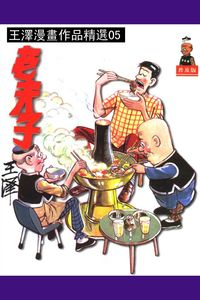### 老夫子. 第5部

• 點閱：9
• 其他題名：漫畫老夫子精選集
• 作者：
• 出版年：1998[民87]
• 出版社：王澤
• 出版地：臺北市
• 集叢名：王澤漫畫作品精選
• 附註：著者本名王家禧

##簡介

《王澤漫畫作品精選：老夫子》風靡港、澳、台及東南亞地區，四十餘年經久不衰，至今總銷量已達六千萬冊以上，堪稱華人漫畫中的經典。

“老夫子”漫畫人物創於1962年。1964年，首期“老夫子”漫畫單行本出版於香港。60年代至70年代，“老夫子”漫畫連載於香港多類報章與雜誌。

《王澤漫畫作品精選：老夫子》並非 ​​完全走寫實諷刺路線。王澤的天馬行空、時空轉移、瘋狂搞笑，更能夠抓緊讀者。有點好色的老父子，可愛憨厚的大番薯，英俊瀟灑的秦先生，三個小人物紮根香港大社會，通過幽默的言行舉止凸現世相萬千，這就是華人傳統漫畫經典《老夫子》中人人樂道的三位主角。

##章節

• 君子好求(p.3)
• 求婚奇遇(p.8)
• 答覆(p.13)
• 愛的魔力(p.18)
• 得意忘形(p.25)
• 老飛(p.30)
• 出風頭(p.35)
• 聖誕禮物(p.40)
• 打桌球(p.48)
• 賣危樓(p.55)
• 粒鈕(p.60)
• 一物兩用(p.65)
• 師生戀(p.70)
• 忍無可忍(p.75)
• 自大狂(p.84)
• 烏龍主筆(p.89)
• 環境與煙(p.94)
• 愛帽如命(p.99)
• 維他命丸(p.104)
• 一分錢一分貨(p.109)
• 神經打電話(p.114)
• 劈材(p.119)
• 保險雪櫃(p.124)
• 對牛彈琴(p.129)
• 烏龍寄信(p.134)
• 避車英雄(p.139)
• 搭車(p.144)
• 順風開遮(p.149)
• 喬遷之喜(p.154)
• 危險獎(p.159)
• 衣服變形記(p.164)
• 烏龍救人(p.169)
• 童心未盡(p.176)
• 為善最樂(p.181)
• 弄巧反拙(p.186)
• 搵苦來辛(p.191)
• 借力(p.196)
• 物極必反(p.201)
• 冒牌英雄(p.206)
• 爆冷門(p.211)
• 沖力不夠(p.216)
• 武功不純(p.221)
• 英雄末路(p.226)
• 英雄末路(p.231)
• 冒牌英雄(p.236)
• 乒乓頭(p.241)
• 原來如此(p.246)
• 自作自受(p.251)
• 一時不慎(p.256)
• 弄巧反拙(p.261)
• 避債一法(p.266)
• 懲狠計(p.271)
• 假道義(p.276)
• 柔能克鋼(p.281)
• 英雄氣短(p.286)
• 喜怒無常(p.291)
• 人猿(p.296)
• 無動於衷(p.301)
• 門當戶對(p.306)
• 女人的魔力(p.311)
• 烏龍送禮(p.316)
• 書迷(p.321)
• 卷毛線(p.326)
• 別有用意(p.331)
• 烏龍拖友(p.336)
• 加料飲品(p.341)
• 分層出售(p.346)
• 大煞風景(p.351)
• 化悲憤為力量(p.356)
• 看破紅塵(p.361)
• 打者愛也(p.366)
• 愛情不專(p.371)
• 談話藝術(p.376)
• 條件問題(p.383)
• 大煞風景(p.388)
• 狂人餐(p.393)
• 委曲求全(p.398)
• 淨冷飲(p.403)
• 虛讓(p.408)
• 虛驚(p.413)
• 快餐(p.421)
• 無動於衷(p.426)
• 劍膽琴心(p.431)
• 心有餘而力不足(p.439)
• 一樣(p.444)
• 阿Q作風(p.449)
• 不知自量(p.454)
• 不知自量(p.454)
• 視死如歸(p.459)
• 變形記(p.464)
• 化敵為友(p.469)
• 虛有其表(p.474)
• 發神經(p.479)
• 勒索驚魂(p.484)
• 原則不變(p.489)
• 啞仔吃黃蓮(p.494)
• 一九六五(p.499)
• 聖誕禮物(p.504)
• 多情的聖誕老人(p.509)
• 一網成擒(p.514)
• 聖誕風雲(p.519)
• 武裝禮物(p.524)
• 得意忘形(p.529)
• 第二年(p.534)
• 放假(p.539)
• 樂極生悲(p.544)
• 真正扭腰舞(p.549)
• 真正阿飛舞(p.554)
• 不知自量(p.559)
• 有樣學樣(p.564)
• 烏龍近視(p.569)
• 表錯情(p.578)
• 英雄氣短(p.583)
• 樹上雨(p.588)
• 隱身術(p.593)
• 跳樓貨(p.598)
• 便宜貨(p.603)
• 一舉兩得(p.608)
• 如此英雄(p.613)
• 打狗看主人(p.618)
• 半斤八兩(p.623)
• 互借(p.628)
• 發神經(p.633)
• 得寸進尺(p.638)
• 對等(p.643)
• 唾汙武器(p.648)
• 掃灰水妙法(p.655)
• 一滴未飲(p.660)
• 徹底(p.665)
• 原則不變(p.670)
• 泄憤(p.675)
• 徒勞無功(p.680)
• 應得報酬(p.685)
• 新襪太緊(p.690)
• 都不知道(p.695)
• 不歡而散(p.700)
• 作狀(p.705)
• 飲多一次(p.710)
• 舊樣翻新(p.715)
• 智取蘋果(p.720)
• 頭獎之災(p.725)
• 另有文章(p.730)
• 一字之錯(p.735)
• 聽電影(p.740)
• 樂善好施(p.745)
• 死不要臉(p.750)
• 烏龍修車(p.755)
• 戲無益(p.760)
• 用力過分(p.765)
• 讀者上當(p.770)
• 大材小用(p.775)
• 並非寫生(p.780)
• 多嘴之過(p.785)
• 自找苦吃(p.790)
• 當機立斷(p.795)
• 終歸是肥(p.800)
• 無能大俠(p.805)
• 真正拖大腳(p.810)
• 事實求是(p.819)
• 惡有惡報(p.824)
• 隨機應變(p.829)
• 冤家路窄(p.834)
• 重慘(p.843)
• 亞洲鐵人(p.848)
• 雪花神劍(p.853)
• 寧死不屈(p.858)